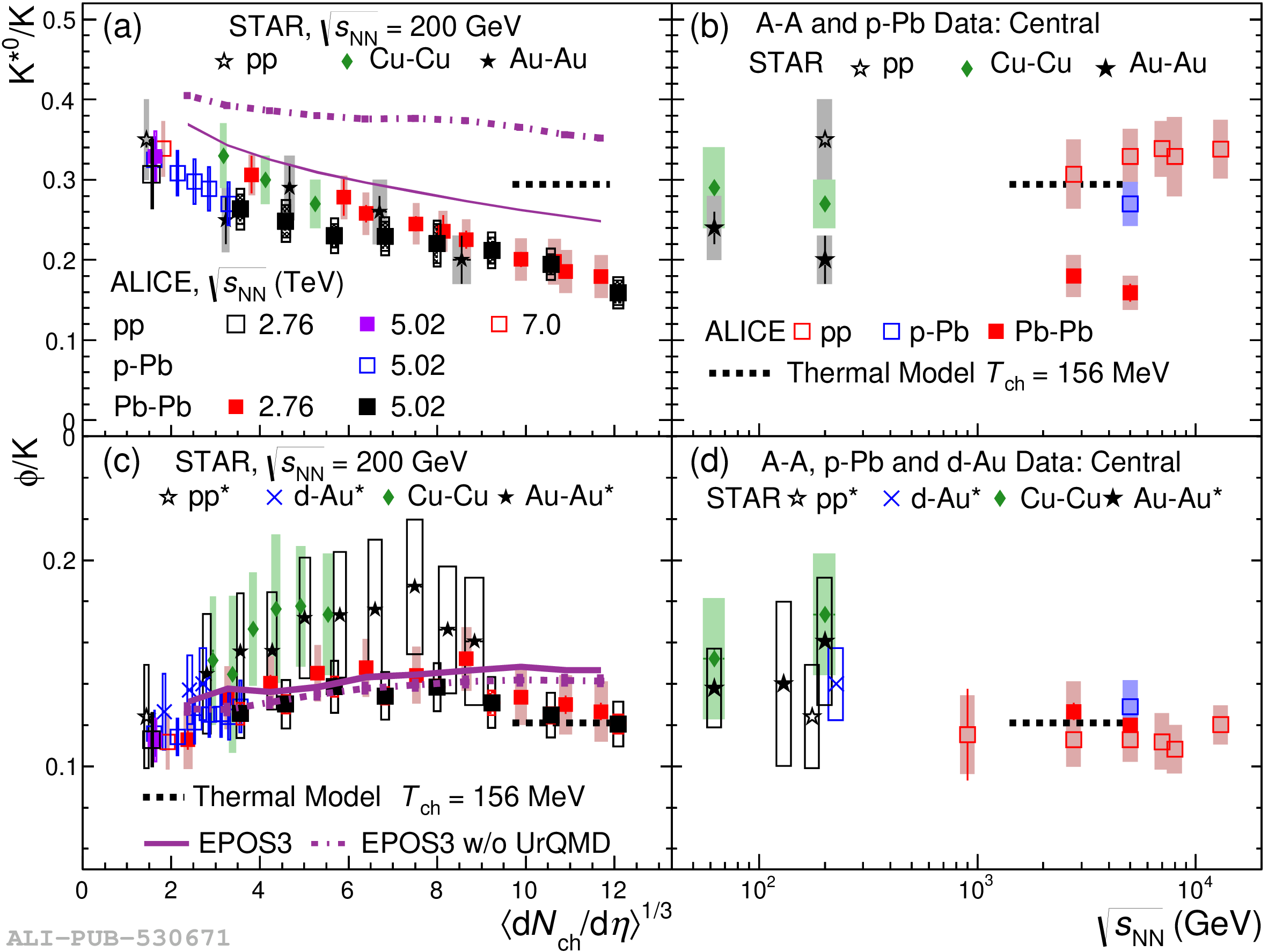# Figure 11

 Particle ratios $\mathrm{K^{*0}/K}$ [panels (a) and (b)] and $\mathrm{\phi/K}$ [panels (c) and (d)] in \pp, \dAu, \pPb, \CuCu, \AuAu, and \PbPb collisions . In panels (a) and (c) these ratios are presented as a function of \avdndeta$^{1/3}$. These ratios are measured as \kskm and \phikm in \PbPb collisions at \snn $=$ 2.76 TeV and in STAR experiment. The values of \avdndeta$_{|\eta|\textless0.5}$ were measured at midrapidity In panels (b) and (d), these ratios are presented for \pp, central \dAu, \pPb, and heavy-ion collisions as a function of \snn. The values given by a grand-canonical thermal model with a chemical freezeout temperature of 156 MeV are also shown . For quantities marked *", boxes represent the total uncertainty (separate uncertainties are not reported). Otherwise, bars represent the statistical uncertainties and boxes represent the systematic uncertainties (including centrality-uncorrelated and centrality-correlated components). EPOS3 model predictions  of $\mathrm{K^{*0}/K}$ and $\mathrm{\phi/K}$ ratios in \PbPb collisions are also shown as violet lines.